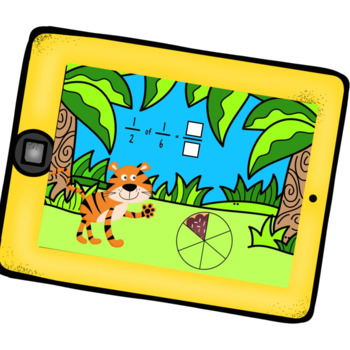# Introduction To Multiplying Fractions Using Google Slides and a Read aloud3rd - 5th, Homeschool
Subjects
Standards
Resource Type
Formats Included
• PDF
Pages
30 pages

### Description

This product is designed to be used after reading "The Lion's Share".

The first set of Google slides shows children a sliced cake and then has them cut the slices in half to figure out what 1/2 of a fraction is. (1/2 of 1/2) (1/2 of 1/3)

The second set has children figuring out what is 1/2 of 2 whole cakes or 1/4 of 3 whole cakes.

Total Pages
30 pages
Not Included
Teaching Duration
40 minutes
Report this Resource to TpT
Reported resources will be reviewed by our team. Report this resource to let us know if this resource violates TpT’s content guidelines.

### Standards

to see state-specific standards (only available in the US).
Apply and extend previous understandings of multiplication to multiply a fraction or whole number by a fraction.
Apply and extend previous understandings of multiplication to multiply a fraction by a whole number.
Decompose a fraction into a sum of fractions with the same denominator in more than one way, recording each decomposition by an equation. Justify decompositions, e.g., by using a visual fraction model. Examples: 3/8 = 1/8 + 1/8 + 1/8; 3/8 = 1/8 + 2/8; 2 1/8 = 1 + 1 + 1/8 = 8/8 + 8/8 + 1/8.
Understand addition and subtraction of fractions as joining and separating parts referring to the same whole.